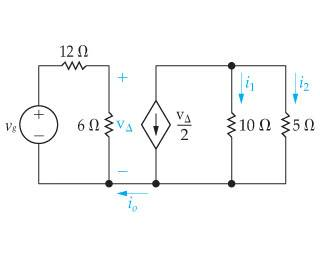# Circuit Analysis KCL question

• Engineering
• ElijahRockers
In summary, the conversation discusses finding the values of i_0, i_1, and i_2 given V_g = 54V. The attempt at a solution involves analyzing the leftmost closed loop and using KCL to determine the current at a node. However, there is confusion about the current flow and the possibility of all currents being equal to zero. It is also noted that the 10Ω and 5Ω resistors are in parallel and that the current source is producing 9A.

Gold Member

## Homework Statementif $V_g = 54V$

find $i_0, i_1, i_2$

## The Attempt at a Solution

In the left most closed loop, Since the source voltage is 54, I assumed there must be a voltage drop across the 12Ω and 6Ω resistors equal to 54. This makes VΔ 18V.

Next I tried to KCL the node below it, but it immediately confused me. If the current around the closed loop is 3A (which is what I calculated by doing a KVL of that leftmost loop), then how can there be any more current flowing into that node? Shouldn't i0 be 0?

Then, even if it is, that means in the righthand part of the circuit, one node has three currents leaving it and none coming in, while another node will have three currents entering it and none leaving. The only way I can think of that happening is if all the currents are equal to zero, but surely this is not the answer...

Anyway, the only other work I could do was to note that the 10Ω and 5Ω are in parallel, so i2 = 2i1. Also, the current source is producing 9A, since the supplied current is supposed to be VΔ/2, but that doesn't seem to help my situation much.

Shouldn't i0 be 0?
Yep! There is nowhere for the current to return...

one node has three currents leaving it and none coming in
That's just a sign thing. Currents can be negative.

i2 = 2i1. Also, the current source is producing 9A

You are on the right track -- keep going!